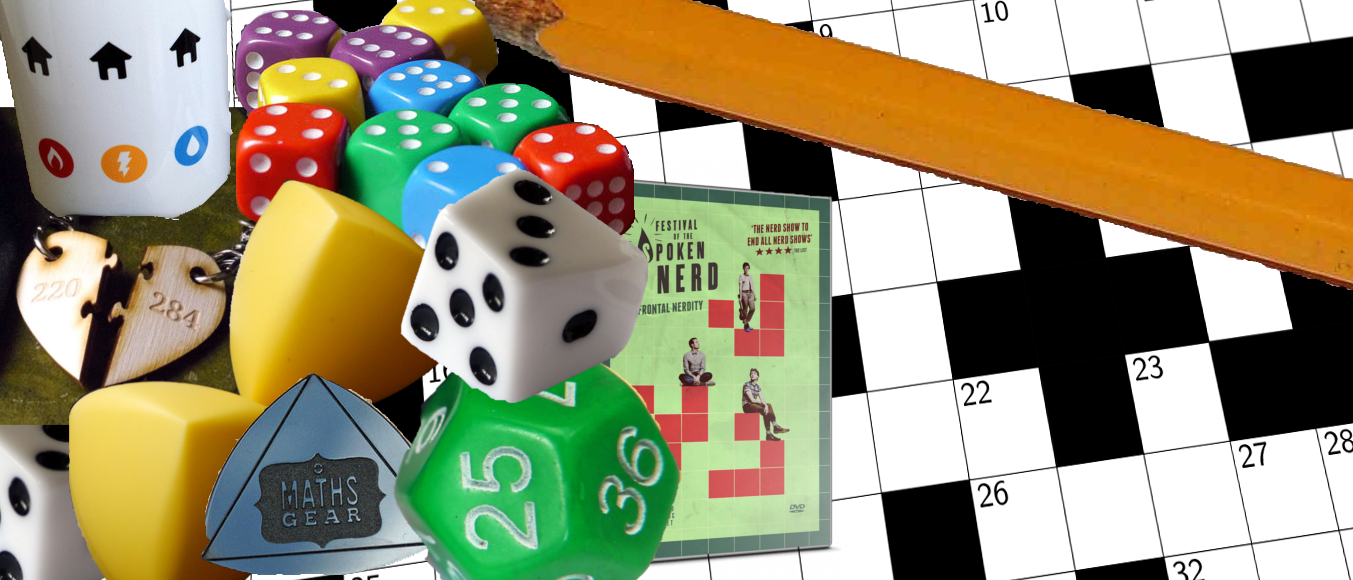# Prize crossnumber, Issue 03

Be in for a chance to win a £100 goody bag if you can solve our fiendish crossnumberOur original prize crossnumber is featured on pages 44 and 45 of Issue 03.

Clarification: In 39D, the integers should be positive and distinct.
Clarification: The answer to 40A is not 5362, as some websites claim. 5362 is the number of positions that could face you after 3 moves, not the number of ways to play 3 moves.

### Rules

• Although many of the clues have multiple answers, there is only one solution to the completed crossnumber. As usual, no numbers begin with 0. Use of Python, OEIS, Wikipedia, etc. is advised for some of the clues.
• One randomly selected correct answer will win a £100 Maths Gear goody bag. Three randomly selected runners up will win a Chalkdust t-shirt. The prizes have been provided by Maths Gear, a website that sells nerdy things worldwide, with free UK shiping. Find out more at mathsgear.co.uk
• To enter, submit the sum of the across clues via this form by 22 July 2016. Only one entry per person will be accepted. Winners will be notified by email and announced on our blog by 30 July 2016.

### Crossnumber

Crossnumber #3, set by Humbug:

### Clues

#### Across

• 1. A multiple of 999. (7)
• 5. Half the difference between 45A and 1A. (7)
• 7. An integer. (3)
• 9. A multiple of 41A. (8)
• 12. 4D multiplied by 43D. (4)
• 13. 43D less than 7A. (3)
• 14. 37A less than 21D. (3)
• 15. A number whose name includes all five vowels exactly once. (5)
• 16. The sum of the digits of 8D. (2)
• 18. An anagram of 24,680. (5)
• 21. A non-prime number whose highest common factor with 756 is 1. (3)
• 24. The sum of the digits of 29D. (2)
• 25. The product of four consecutive Fibonacci numbers. (6)
• 26. The product of 14A and 21D. (6)
• 29. The largest known $n$ such that all the digits of $2^n$ are not zero. (2)
• 30. The HTTP error code for “I’m a teapot”. (3)
• 32. A prime number that is the sum of 25 consecutive prime numbers. (5)
• 35. The number of different nets of a cube (with reflections and rotations being considered as the same net). (2)
• 36. A Fibonacci number. (5)
• 37. When written in a base other than 10, this number is 256. (3)
• 39. Why is 6 afraid of 7? (3)
• 40. The number of ways to play the first 3 moves (2 white moves, 1 black move) in a game of chess. (4)
• 41. A multiple of 719. (8)
• 42. A prime number that is two less than another prime number. (3)
• 44. Half of 29D. (7)
• 45. The sum of 6D, 8D, 31D, 37D and 43D. (7)

#### Down

• 1. A power of 2. (7)
• 2. A palindrome. (8)
• 3. This number’s cube root is equal to its number of factors. (5)
• 4. An odd number. (2)
• 5. A square number. (5)
• 6. 5D multiplied by 1 less than 27D. (7)
• 8. An odd number. (6)
• 10. A multiple of 7. (2)
• 11. The number of pairs of twin primes less than 1,000,000. (4)
• 17. The number of factors of 26A. (3)
• 19. Greater than 30A. (3)
• 20. Each digit of this number is a prime number and larger than the digit before it. (3)
• 21. 37A more than 14A. (3)
• 22. A multiple of 27. (3)
• 23. A number $n$ such that $(n-1)!+1$ is divisible by $n\hspace{1pt}^2$. (3)
• 24. The smallest number that cannot be changed into a prime by changing one digit. (3)
• 27. A three digit number. (3)
• 28. A multiple of 34D. (8)
• 29. A multiple of 7. (7)
• 31. A prime number in which three different digits each appear twice. (6)
• 33. 1,000,006 less than 6D. (7)
• 34. The smallest number that is non-palindromic when written in binary, but whose square is palindromic when written in binary. (4)
• 37. The square of this number only contains the digits 1, 2, 3 and 4. (5)
• 38. A cube number. (5)
• 39. The largest number that cannot be written as the sum of positive, distinct integers, the sum of whose reciprocals is 1. (2)
• 43. The smallest number that is twice the sum of its digits. (2)